# Understanding HSVL - Guillermo Algora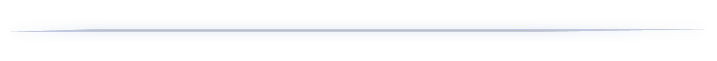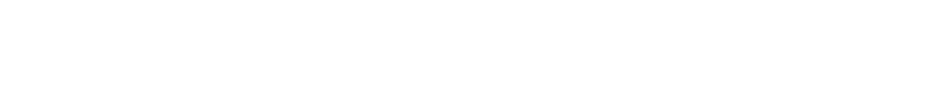Guillermo Algora
Go to content
UNDERSTANDING HSVL
WITHIN THE NUKE CONTEXT

1. HUE:

Hue is an attribute to classify colours based on its dominant wavelength, and with independence of intensity (saturation) or lightness (luminance). Hues are usually represented in a 360° colorwheel (as in Nuke) or in a spectrum bar.
There are 12 main hues in a color wheel: red, orange, yellow, chartreuse green, green, spring green, cyan, azure, blue, violet, magenta and rose. Each of them every 30°.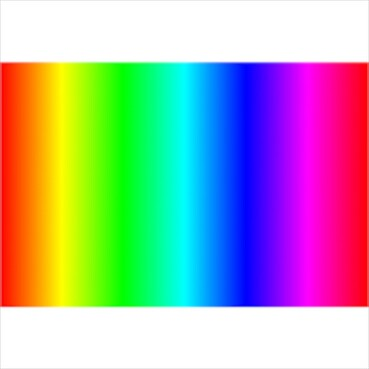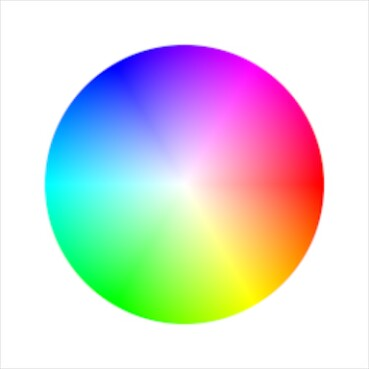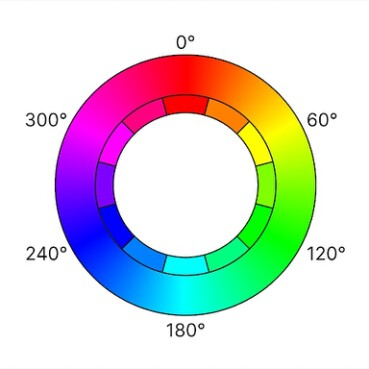The Hue value represents the angle on the colour wheel. In Nuke, we can find the hue angle of the sampled area displayed at the bottom right corner of the Viewer Panel. The interesting part about the Hue value is that it is (as mentioned above) independent of Saturation or Brightness. This pecuarility makes it really useful when matching two different elements. We could certainly grade our element by eye until both of the Hue values match, however, to do it more efficiently, I propose the following method:

We will convert our element to the HSL (equally possible the HSV) Colorspace. This Colorspace maps the Hue, Saturation and Luminance values of the image into the red, green and blue channel respectively. We now can modify the Hue value
(rotate around the colour wheel) by increasing/reducing the value in the red channel. We can also increase/reduce Saturation by changing the green channel, and the same for Luminance in the blue channel. We can make use of a Grade Node and tick the channel that we want to modify, in this case red . I recommend to use the Offset function, as it is purely adding / subtracting value.

(Check, high saturation two channels?)
Now, let us have look at how the Hue value is calculated.

• Identify the maximum (max) and minimum (min) of red, green and blue values.

• If the values of the three channels are the same or max = 0:
Hue is zero (the colour posses 0 saturation). However, keep in mind that also pure red is represented with a Hue value of 0.

• If red = max:
Hue = 60 * ((green - blue) / (max - min))

• If green = max:
Hue = 60 * (2.0 + (blue - red) / (max - min))

• If blue = max:
Hue = 60 * (4.0 + (red - green) / (max - min))
2. SATURATION:

Saturation is independent of Hue or Luminace and is an indicator of the intensity of a colour. Highly saturated colours appear "stronger", although increasing Saturation also reveals more of the Hue present in the colour, for example being 'more red', 'more blue', etc. That is because, while zero Saturation (i.e. grey) means that all three channels have the same value (R=G=B, e.g. 0.5, 0.5, 0.5) and low Saturation means that the three channels (red, green, blue) have close values, high Saturation represents a strong disparity among the values of the three channels. Increasing Saturation pulls the values in the channels further apart, thus increasing the dominant Hue.

Let us have a look at how the Saturation value (displayed) within the Nuke Viewer Panel is calculated:

• Identify the maximum (max) and minimum (min) of red, green and blue values.

• Saturation = max - min / max

And now, let us take a look at the four saturation luminance methods within the Nuke's Saturation node:

First, Nuke desaturates the image using the desired luminance maths (as chosen in the 'math dropdown' within the node):

There are four methods: Rec.709 (which is also the method for calculating the Luminance parameter in the Viewer Panel), Ccir 601, Average and Maximum (the method for calculating the 'Value' parameter in the Viewer Panel). And their maths:

REC. 709:
Luminace = R * 0.2126 + G * 0.7152 + B * 0.0722

CCIR 601
Luminance = R * 0.299 + G * 0.587 + B * 0.114

AVERAGE
Luminance = (R + G + B) / 3

MAXIMUM
Luminance = Max(R, G, B)

Once that is completed, then does a linear interpolation (lerp) between the Luminance value (a) and the original value (b) with the attribute of the node's saturation value (t).

ouput value = lerp (a, b, t)

When t  is 0, the function returns the value of a (full desaturation).
When t  is 1, it returns the value of b (original value).
Otherwise, it returns a value in between at the t  point (Saturation value) between the two.

In other words, what desaturation within Nuke means is a conversion to luminance (with different luminance maths) and a mix with the original image.
3. VALUE:

Value in Nuke's Viewer Panel is the simplest of all as it represents the highest from the three channels: R, G or B.

4. LUMINANCE:

L in the Nuke's Viewer Panel stands for luminance, computed with the rec709 coefficients (a weighted sum of red green and blue):

L = R * 0.2126 + G * 0.7152*G + B * 0.0722

In other words, the Luminance is rather property of the image in linear color space, whilst the Value is a property of the image displayed in the viewer, according to the HSV color model.

I misspoke. So there are two values in the bottom right of the viewer. The left most one is always in scene linear values and the right side one is the 8 bit value or whatever option you pick in the down arrow drop down. (left side he refers to the RGB SAMPLE, the other whatever option you pick from the dropdown.

Dropdown

HSVTool

why coefficients
increase saturation/ what happens
negative values when increasing saturation
Back to content